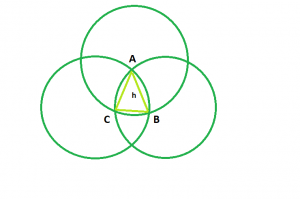# Area of Reuleaux Triangle

Given an integer h which is the side of an equilateral triangle formed by the same vertices as the Reuleaux triangle, the task is to find and print the area of the Reuleaux triangle.Examples:

Input: h = 6
Output: 25.3717

Input: h = 9
Output: 57.0864

## Recommended: Please try your approach on {IDE} first, before moving on to the solution.

Approach: The shape made by the intersection of the three circles ABC is the Reuleaux Triangle and the triangle formed by the same vertices i.e. ABC is an equilateral triangle with side h.

Now, Area of sector ACB, A1 = (π * h2) / 6
Similarly, Area of sector CBA, A2 = (π * h2) / 6
And, Area of sector BAC, A3 = (π * h2) / 6
So, A1 + A2 + A3 = (π * h2) / 2
Since, the area of the triangle is added thrice in the sum.
So, Area of the Reuleaux Triangle, A = (π * h2) / 2 – 2 * (Area of equilateral triangle) = (π – √3) * h2 / 2 = 0.70477 * h2

Below is the implementation of the above approach:

## C++

 `// C++ Program to find the area ` `// of Reuleaux triangle ` `#include ` `using` `namespace` `std; ` ` `  `// Function to find the Area ` `// of the Reuleaux triangle ` `float` `ReuleauxArea(``float` `a) ` `{ ` ` `  `    ``// Side cannot be negative ` `    ``if` `(a < 0) ` `        ``return` `-1; ` ` `  `    ``// Area of the Reuleaux triangle ` `    ``float` `A = 0.70477 * ``pow``(a, 2); ` `    ``return` `A; ` `} ` ` `  `// Driver code ` `int` `main() ` `{ ` `    ``float` `a = 6; ` `    ``cout << ReuleauxArea(a) << endl; ` `    ``return` `0; ` `} `

## Java

 `// Java Program to find the area  ` `// of Reuleaux triangle  ` ` `  `public` `class` `GFG ` `{ ` `    ``// Function to find the Area  ` `    ``// of the Reuleaux triangle  ` `    ``static` `double` `ReuleauxArea(``float` `a)  ` `    ``{  ` `     `  `        ``// Side cannot be negative  ` `        ``if` `(a < ``0``)  ` `            ``return` `-``1``;  ` `     `  `        ``// Area of the Reuleaux triangle  ` `        ``double` `A = (``double``)``0.70477` `* Math.pow(a, ``2``);  ` `        ``return` `A;  ` `    ``}  ` `     `  `    ``// Driver code  ` `    ``public` `static` `void` `main(String args[])  ` `    ``{  ` `        ``float` `a = ``6``;  ` `        ``System.out.println(ReuleauxArea(a)) ;  ` `    ``}  ` `    ``// This code is contributed by Ryuga ` `} `

## Python3

 `# Python3 program to find the area ` `# of Reuleaux triangle ` `import` `math as mt ` ` `  `# function to the area of the  ` `# Reuleaux triangle ` `def` `ReuleauxArea(a): ` `     `  `    ``# Side cannot be negative ` `    ``if` `a < ``0``: ` `        ``return` `-``1` `    ``# Area of the Reauleax triangle ` `    ``return` `0.70477` `*` `pow``(a, ``2``) ` ` `  `# Driver code ` `a ``=` `6` `print``(ReuleauxArea(a)) ` ` `  `# This code is contributed  ` `# by Mohit Kumar         `

## C#

 `// C# Program to find the area   ` `// of Reuleaux triangle   ` `   `  `using` `System; ` ` `  `public` `class` `GFG  ` `{  ` `    ``// Function to find the Area   ` `    ``// of the Reuleaux triangle   ` `    ``static` `double` `ReuleauxArea(``float` `a)   ` `    ``{   ` `       `  `        ``// Side cannot be negative   ` `        ``if` `(a < 0)   ` `            ``return` `-1;   ` `       `  `        ``// Area of the Reuleaux triangle   ` `        ``double` `A = (``double``)0.70477 * Math.Pow(a, 2);   ` `        ``return` `A;   ` `    ``}   ` `       `  `    ``// Driver code   ` `    ``public` `static` `void` `Main()   ` `    ``{   ` `        ``float` `a = 6;   ` `        ``Console.WriteLine(ReuleauxArea(a)) ;   ` `    ``}  ` `} ` `    ``// This code is contributed by Subhadeep `

## PHP

 `

Output:

```25.3717
```

Attention reader! Don’t stop learning now. Get hold of all the important DSA concepts with the DSA Self Paced Course at a student-friendly price and become industry ready.

My Personal Notes arrow_drop_upCheck out this Author's contributed articles.

If you like GeeksforGeeks and would like to contribute, you can also write an article using contribute.geeksforgeeks.org or mail your article to contribute@geeksforgeeks.org. See your article appearing on the GeeksforGeeks main page and help other Geeks.

Please Improve this article if you find anything incorrect by clicking on the "Improve Article" button below.